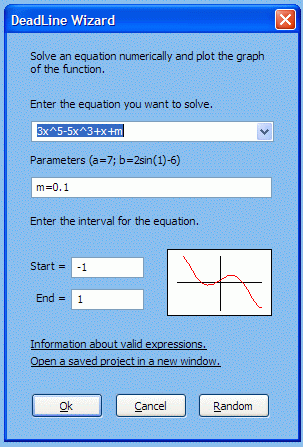Useful Hacks, Reviews, Tips and Tricks of anything under the moon and the sun.

# Solve Math Equations Using Deadline

During my school days, when it comes to solving Math problems, my best friend is my complicated scientific calculator. In today's age, it is a lot easier for engineering and students to solve math equations in seconds by using one of plenty tools available on the internet. One application that deserves mentioning is Deadline. It is a freeware made for students and engineers. It combines graph plotting with advanced numerical Calculus, in a very intuitive approach. Most equations are supported, including algebraic equations, trigonometric equations, and exponential equations.- To solve equations
- Plot graphs interactively
- Perform calculations
- Derive functions and evaluate derivatives
- Solve optimization problems: find local extrema
- Integrate functions numerically
- Solve parametric equations
- Obtain an in-depth analysis of a function
- Save results: equations, graphs, roots and extrema
- Solve Calculus assignments in your own language